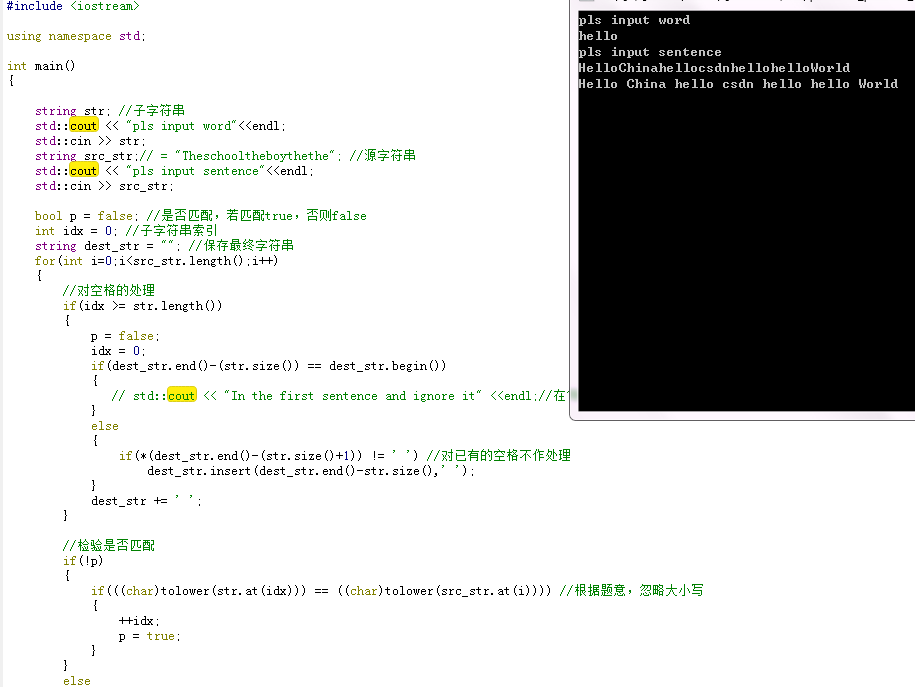【问题描述】

【输入形式】

【输出形式】

【输入样例】
the
Theschooltheboythethe
【输出样例】
The school the boy the the
【样例说明】

2个回答``````#include <iostream>

using namespace std;

int main()
{

string str; //子字符串
std::cout << "pls input word"<<endl;
std::cin >> str;
string src_str;// = "Theschooltheboythethe"; //源字符串
std::cout << "pls input sentence"<<endl;
std::cin >> src_str;

bool p = false; //是否匹配，若匹配true，否则false
int idx = 0; //子字符串索引
string dest_str = ""; //保存最终字符串
for(int i=0;i<src_str.length();i++)
{
//对空格的处理
if(idx >= str.length())
{
p = false;
idx = 0;
if(dest_str.end()-(str.size()) == dest_str.begin())
{
// std::cout << "In the first sentence and ignore it" <<endl;//在句子首位忽略它
}
else
{
if(*(dest_str.end()-(str.size()+1)) != ' ') //对已有的空格不作处理
dest_str.insert(dest_str.end()-str.size(),' ');
}
dest_str += ' ';
}

//检验是否匹配
if(!p)
{
if(((char)tolower(str.at(idx))) == ((char)tolower(src_str.at(i)))) //根据题意，忽略大小写
{
++idx;
p = true;
}
}
else
{
if(((char)tolower(str.at(idx))) == ((char)tolower(src_str.at(i)))) //根据题意，忽略大小写
{
++idx;
p = true;
}
else
{
p = false;
idx = 0;
}
}
dest_str += src_str.at(i);
}
std::cout << dest_str << std::endl;

return 0;
}
``````10 个月之前 回复qtchen_1988 回复狮子歌歌丶: 你用什么编译的，我已经改了，可以输入的，而且是尽量符合一般编译器的
10 个月之前 回复10 个月之前 回复10 个月之前 回复